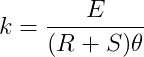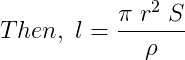Conversion of Galvanometer to Ammeter

# Our Objective:

To convert the given galvanometer (of known resistance and figure of merit) into an ammeter of desired range and to verify the same.

# The Theory:

## What is a Galvanometer?

A galvanometer is a device used to detect feeble electric currents in a circuit.  It has a coil pivoted (or suspended) between concave pole faces of a strong laminated horse shoe magnet.  When an electric current passes through the coil, it deflects.   The deflection is proportional to the current passed. The galvanometer coil has a moderate resistance (about 100 ohms) and the galvanometer itself has a small current carrying capacity (1 mA).

## What is an Ammeter?

An ammeter is a device used for measuring large electric currents in circuits.  For this purpose, it is put in series with the circuit in which the current is to be measured.

## How to convert a Galvanometer into an Ammeter?

A galvanometer can detect only small currents.  Thus, to measure large currents it is converted into an ammeter.  It can be converted into an ammeter by connecting a low resistance called shunt resistance in parallel to the galvanometer.

Let G be the resistance of the galvanometer and Ig be the current for full scale deflection in the galvanometer, the value of the shunt resistance required to convert the galvanometer into an ammeter of 0 to I ampere is,

$S=\frac{{I}_{g}×G}{I-{I}_{g}}$

Ig is calculated using the equation, Ig = nk, where n is the number of divisions on the galvanometer and k is the figure of merit of galvanometer.

## What is figure of merit of a galvanometer?

The figure of merit of a galvanometer is defined as the current required in producing a unit deflection in the scale of the galvanometer.  It is represented by the symbol k and is given by the equation,Where E is the e.m.f. of the cell and θ is the deflection produced with resistance R.

Let ‘l’ be the length of the resistance wire required for a resistance of S ohm,where, r is the radius of the wire and ρ is the resistivity of the material of the wire.

# Learning Outcomes:

The student learns the following concepts:

• Galvanometer
• Ammeter
• Figure of merit
• Shunt resistance
• How a galvanometer can be converted into an ammeter.